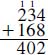# What does carry mean in addition?

In addition, carry defines a way in which larger numbers are added. In this arithmetic operation, there is a shifting of leading digits into the next column to the left when the sum of that column exceeds a single digit. For example, carrying is evident in the following operation in the base 10 numbering system (adding addends 234 and 168 giving the sum 402):By adding the same columns, starting in the right hand column, 8 + 4 is 12, a number larger than 9, the highest number than can exist in that spot; carry the amount of ten to the next column, leaving 2; add 3 + 6 + 1 (which carried, represents 10 for this column), which equals 10, again a number larger than 9. Again, carry the one to the next column leaving 0; add the 2 + 1 + 1 (carried) equals 4. All total, the sum is 402.

Close

This is a web preview of the "The Handy Math Answer Book" app. Many features only work on your mobile device. If you like what you see, we hope you will consider buying. Get the App